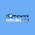# Special Angles in Trigonometry

Some angles in trigonometry are so common, they are known as special angles.  The values of trig functions of these specific angles can be represented by known ratios, and are good to commit to memory to help you work through problems faster. These angles can be remembered by examining two different special angle triangles. Specifically, the trig functions for angles of 0, 30, 45, 60, 90 degrees are the special ones.  (Please hit the Like button or +1 button at the end if you think this post helps you with special angles!)

Let's look at the first of the special angle triangles, and this will hopefully become clear. Take a right angle triangle with two 45 degree angles, and with sides of 1 unit length. By the Theorem of Pythagoras, the hypotenuse of this triangle is of length √2. This is what this triangle looks like:
So then, from these values and SOHCAHTOA, you can obtain the trig values for this special angle of 45 degrees. You can see that:

Sin(45) = 1/√2
Cos(45) = 1/√2
Tan(45) = 1

Don't worry if you can't remember these exact ratios... the simplest thing to remember is how to construct the special angle triangle... which is as easy as remembering a right angle triangle with a 45 degree angle and 2 sides of length 1... you can easily fill in the rest, and then work out the trig ratios yourself!

The second of the special angle triangles, which represents the rest of the special angles to remember, is slightly more complex, but still straightforward. Take a right angle triangle with angles of 30, 60, and 90 degrees. The simplest lengths of the sides of this triangle are 1, 2, √3 (with 2 being the longest side, the hypotenuse). This 30 60 90 triangle looks like this:
So then, using the these values, you can obtain the trig values for these special angles as well:

Sin(30) = 1/2
Cos(30) = √3/2
Tan(30) = 1/√3

Sin(60) = √3/2
Cos(60) = 1/2
Tan(60) = √3/1 = √3

Again, just remember how to construct the triangle, and the ratios are easy to come up with!

For 0 and 90 degrees, there isn't a simple triangle scheme to remember the values (although please feel free to correct me if I am wrong!). However, these aren't scary square root numbers or weird fractions:

Sin(0) = 0
Cos(0) = 1
Tan(0) = 0

Sin(90) = 1
Cos(90) = 0
Tan(90) = undefined

If you can familiarize yourself with all of these special angles, or at least understand how to derive them from the special angle triangles, then you will have a much easier time working on trigonometry questions.  Again, please remember to Like and/or +1 this post if it helped.  Thanks.

#### 16 comments:

1.Remembering these triangles and their respective values has always been a troubling concept for me. Thanks for making it sound like it is doable with just some simple reasoning. Good job sk. =p =)

2.This is one of my favorite blogs. Thanks! How do you get all the cool pictures and the math symbols into the blog?

3.Anonymous:
Thank you, you have saved me from miscomprehension of yes... poor teaching indeed.
@ alexmcferron
He must have constructed the picture using an image editor/maker. Handwork + scanning works well too.

4.Thanks for the comments! I created the graphs with the Microsoft PowerToys Calculator. It has a graphing function, and you can cut and paste the images. The triangles and other images are all made by hand. (I thought I responded to this question a long time ago, but apparently not! Sorry!)

5.I was wondering if showing the relationships of the sides of special triangles without a variable might be clear enough to a beginner.

What happens if the short leg of a 30 45 60 deg triangle is 3? Would a beginner know to multiply 3 by sqrt 3 to get the longer leg?

6.@ Instructivist

I posted a new entry to hopefully address your question...

http://sk19math.blogspot.com/2008/06/special-angles-in-trigonometry-part-2.html

Please let me know what you think. :)

7.I have scoured the internet for a sound explanation of these special values. Thank you, you are a star.

8.man you save me a lot!!! love you!

9.thanks...it helped me a lot for my case study..=)

10.Annyeong,

you know, i really don't understand trigo..but this helps me a lot..when i read this , i can now imagine how easy trigo is..contrary to geom., i will never understand the topics..hehe..

Gamsa Hamnida..

11.Hi. I was wondering how do you work out the area of a triangle when i dont know the values of the angles, but have all the measurements of the sides. The triangles dont have symmetrical values either.

i.e. sides A=310, B=420, C=600

Hope you can help me. Need to work this out for an assignment

12.@Anonymous,

Try using the cosine law to find the first angle... then use the sine law to find the remaining angles.

When you have the angles, you can use the Area = 1/2 base x height formula. Align the triangle with one side horizontal, and draw a line up to the top point. Then with the angles you have solved, you can calculate the height of the triangle.

Give it a try and let me know how it goes. :)

13.can you give some problems in solving special angles? i just want to know. but then, i learn a lot from your explaination. good bless.

14.you teach me a lot.thank you so much.more powers and god bless you.

15.` I hate MATH !! promise .. but despite for that , i challenge my self to conquer this SUBJECT !! coz i realize math subject is NOT ONLY FOR GENIUS PEople !!

But it so very Fun to SOlve The problems !

16.This is so helpful to learn angels issues related to math example. This post is really very effective for students. It describes the rule so easily.